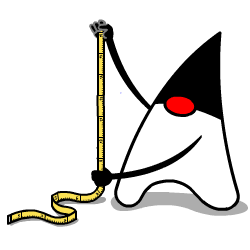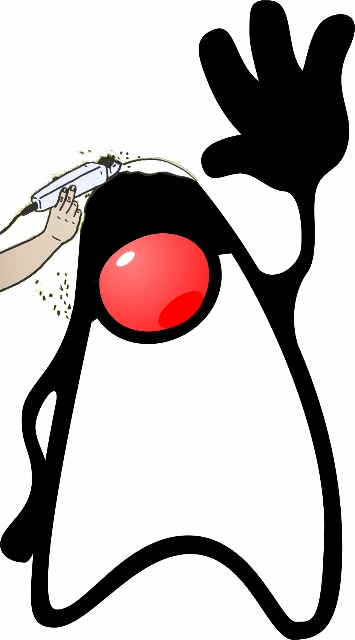The Unit of Measurement API provides a set of Java language programming interfaces for handling units and quantities.
The interfaces provide a layer which separates client code, that would call the API,
from library code, which implements the API.The specification contains Interfaces and abstract classes with methods for unit operations:
* Checking of unit compatibility
* Expression of a quantity in various units
* Arithmetic operations on units

Developers frequently encounter the need to model units of measure, because objects in the real world are subject to these measures.
When working with units, developers need to understand the mathematics of units, how to convert between systems,
and how to format and parse string representations of units. Most of this work can be consolidated into one or two Java packages,
which is a primary aim of this JSR. This package will help developers create safe, correct software to deal with common problem of modeling units.

Units give us a way to measure the physical world. There are many different units, partly because the world has different types of properties,
such as length and mass, which are not interchangeable in normal physics. This type of property is sometimes called a "quantity" or a "dimension".
The word "dimension" fits because of the orthogonality of these properties. For example "mass" and "length" cannot be exchanged.
Further, when we multiply physical measures the dimensions add up as exponents. For example, length times length becomes length².

A large body of work exists that specifies the dimensions, meanings, and names of various physical quantities.
In particular, the 11th General Conference on Weights and Measures in 1960 recommended a practical system of units of measurement,
and gave it the name Systeme International d'Units (SI).
SI defines units for the base dimensions of length, mass, time, electric current,
thermodynamic temperature, amount of substance, and luminous intensity.
SI also recognizes names and meaning of derived dimensions,
such as area, volume, and force.
For some (but not all) of these derived dimensions SI defines units,
such as "liter" for a unit of volume and "newton" for a unit of force.
SI does not have a special name for area,
although other systems of measurement do (as in "acre").

Despite the rising prominence of the metric system (the SI system), many developers have to work with non-SI units,
such as feet, miles, acres, and gallons. A measurement can be expressed as a number of any unit,
so long as the unit has the same dimension as the measured quantity. For example,
any measure of volume can be expressed as a number of liters or gallons,
because liters and gallons are units of volume, and all volumes have the dimension of length^3.
Therefore, a measure expressed as a number of liters can be 'converted' to a number of gallons.
Converting measures from one system of units to another is a common problem,
but is subject to mathematics that can help to eliminate errors.

What's with Duke and that haircut?The image refers to the Shakespeare play "Measure for Measure",
occasionally used by presentations on JSR 363.
In the story a Duke disguised as a friar spies on his subjects, so Duke sounded like a good match (not for the spying we hope;-)